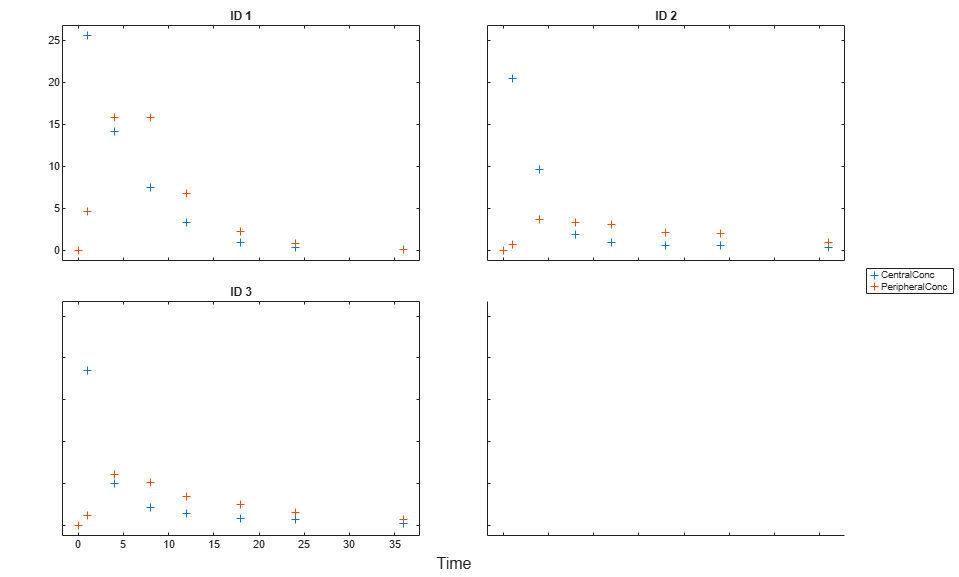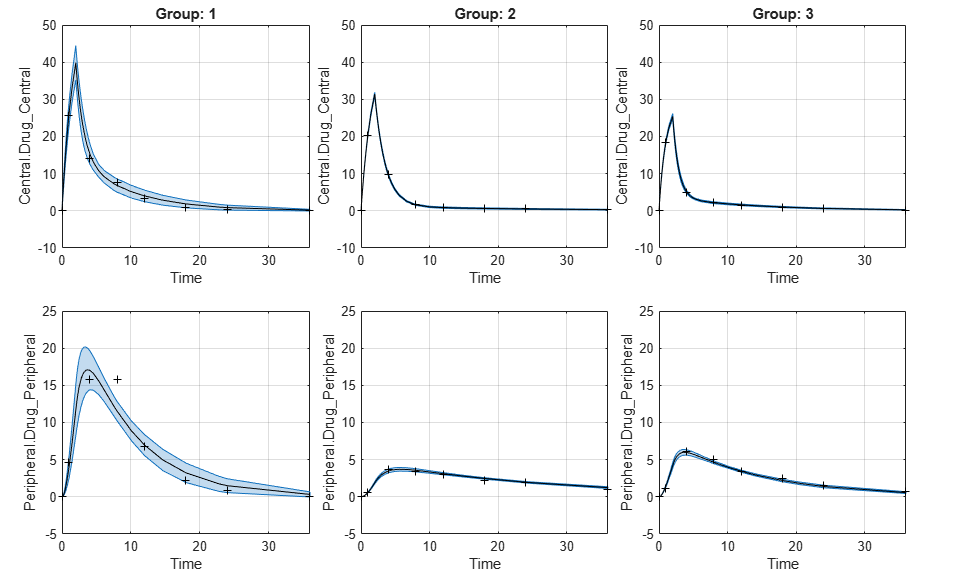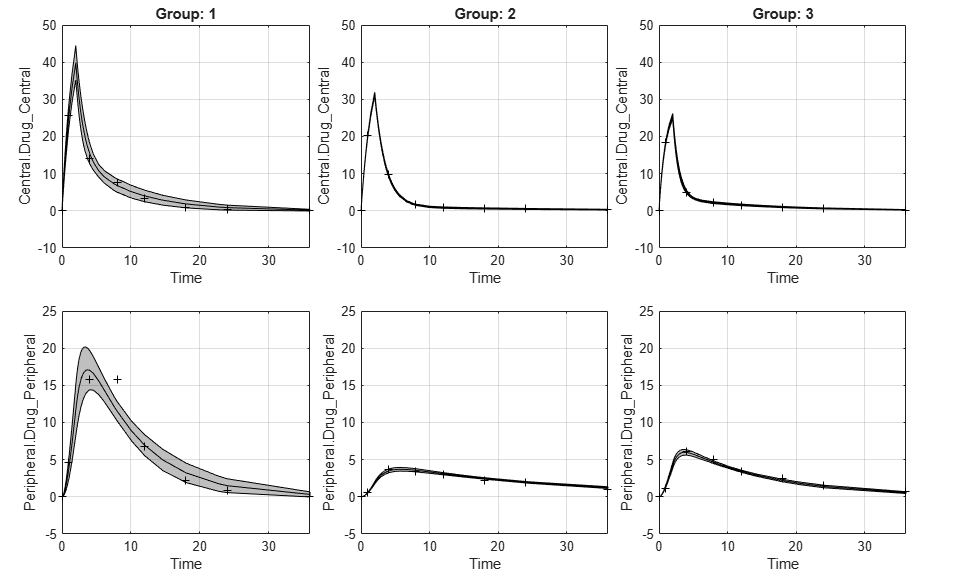plot

Plot confidence interval results for model predictions

Description

example

fh = plot(predCI) plots confidence interval results from predCI, a PredictionConfidenceInterval object or vector of objects.

The function plots the observation data points as black plus signs and the model predictions as solid lines.

• If the status of confidence interval (predCI.Status) is constrained or not estimable, the function uses the second default color (red) to plot the confidence intervals.

• Otherwise, the function uses the first default color (blue) and plots the confidence intervals as shaded areas.

Examples

collapse all

Load the sample data to fit.

gData = groupedData(data);
gData.Properties.VariableUnits = {'','hour','milligram/liter','milligram/liter'};
sbiotrellis(gData,'ID','Time',{'CentralConc','PeripheralConc'},'Marker','+',...
'LineStyle','none');Create Model

Create a two-compartment model.

pkmd                 = PKModelDesign;
pkc1.DosingType      = 'Infusion';
pkc1.EliminationType = 'linear-clearance';
pkc1.HasResponseVariable = true;
model                = construct(pkmd);
configset            = getconfigset(model);
configset.CompileOptions.UnitConversion = true;

Define Dosing

Define the infusion dose.

dose             = sbiodose('dose','TargetName','Drug_Central');
dose.StartTime   = 0;
dose.Amount      = 100;
dose.Rate        = 50;
dose.AmountUnits = 'milligram';
dose.TimeUnits   = 'hour';
dose.RateUnits   = 'milligram/hour';

Define Parameters

Define parameters to estimate.

responseMap = {'Drug_Central = CentralConc','Drug_Peripheral = PeripheralConc'};
paramsToEstimate   = {'log(Central)','log(Peripheral)','Q12','Cl_Central'};
estimatedParam     = estimatedInfo(paramsToEstimate,...
'InitialValue',[1 1 1 1],...
'Bounds',[0.1 3;0.1 10;0 10;0.1 2]);

Fit Model

Perform an unpooled fit, that is, one set of estimated parameters for each patient.

unpooledFit = sbiofit(model,gData,responseMap,estimatedParam,dose,'Pooled',false);

Compute Confidence Intervals for Model Predictions

Compute 95% confidence intervals for predicted model responses in the unpooled fit using the Gaussian approximation.

ciPredUnpooled = sbiopredictionci(unpooledFit);

Plot the confidence intervals. If the estimation status of a confidence interval is constrained or not estimable, the function uses the second default color (red). Otherwise, the function uses the first default color (blue). To see the color order, type get(groot,'defaultAxesColorOrder').

Each group is displayed in each column, from left to right, in the same order that they appear in the GroupNames property of the object, which is used to label each column.

plot(ciPredUnpooled)Plot using a single color (black).

plot(ciPredUnpooled,'Color',[0 0 0])Input Arguments

collapse all

Confidence interval results for model predictions, specified as a PredictionConfidenceInterval object or a vector of objects.

If there are multiple groups, each group is displayed in each column, from left to right, in the same order that they appear in the GroupNames property of the object. Each row represents a model response.

Name-Value Arguments

Specify optional comma-separated pairs of Name,Value arguments. Name is the argument name and Value is the corresponding value. Name must appear inside quotes. You can specify several name and value pair arguments in any order as Name1,Value1,...,NameN,ValueN.

Example: 'Color',[0 0 0] specifies to plot using only the color black.

Red-Green-Blue color triplet, specified as the comma-separated pair consisting of 'Color' and a three-element row vector. By default, confidence intervals that are not limited by parameter bounds specified in the original fit are plotted using the first default color (blue), and those that are limited by the bounds are plotted using the second default color (red). If the confidence interval is not estimable, it is also plotted in red. To see the default color order, enter get(groot,'defaultAxesColorOrder') or see the ColorOrder property.

Tip

Use this name-value pair when you want to create plots with a single color, for instance, for publication purposes.

Example: 'Color',[0 0 0]

Output Arguments

collapse all

Figure handle of the plot, returned as a figure handle.